# Random Velocity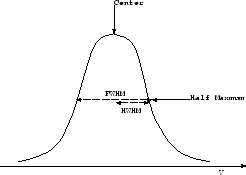Considering gas in Maxwellian velocity distribution, the distribution function for the velocity is as follows: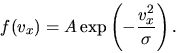(E.1)

This gives the one-dimensional random velocity as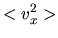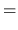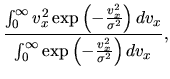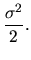(E.2)

If we observe emissions from such a gas, the emission line is broaden due to the Doppler shift. Using equation (E.2), the HWHM (half width of half maximum: the line width measured from the the center of the emission line to the point of the half intensity; see Fig.E.1) of the emission line is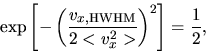(E.3)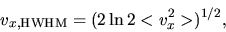(E.4)

and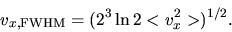(E.5)

Thus, if we assume isotropic distribution, three-dimensional random velocity of gas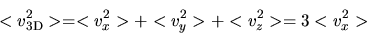(E.6)

is obtained with the line width as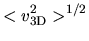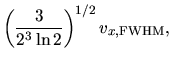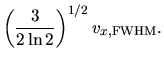(E.7)